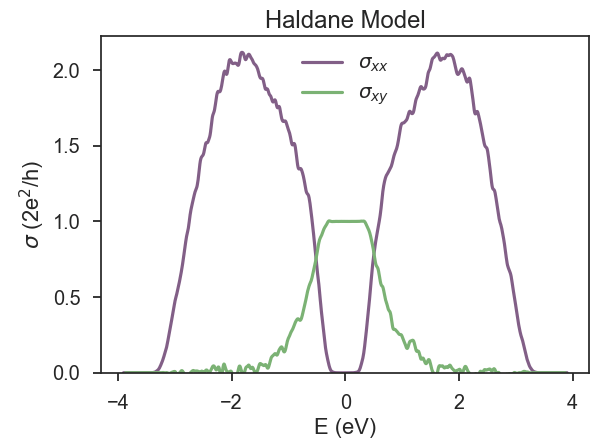# Disordered Haldane model and the transverse conductivity

Haldane Hamiltonian is a single-orbital tight-binding model on a honeycomb lattice with a sublattice-staggered on-site potential (orbital mass) and complex hoppings between next-nearest-neighbor sites that produce a staggered magnetic field configuration with vanishing total flux through the unit cell 1. This model is a Chern insulator (or a quantum anomalous Hall insulator because it hosts integer quantum Hall effect in the absence of an external magnetic field). This characteristic makes Haldane model ideal for illustrating another capability of KITE: the calculation of transverse conductivities.

Let us begin with the definition of the Hamiltonian for the case of pure imaginary next-nearest-neighbor hoppings:

``````def haldane():
"""Return the lattice specification for haldane model"""
a = 0.24595 #lattice constant
a_cc = a/sqrt(3)  #distance between neighbors
t = -1      #  nearest neighbour hopping
t2 = 0.1t/    #strength of the complex hopping
m=0.1 #orbital mass
# create a lattice with 2 primitive vectors
lat = pb.Lattice(
a1=[a, 0],
a2=[a/2, a/2 * sqrt(3)]
)

# name and position
('A', [0, -a_cc/2],m), #onsite potential (orbital mass)
('B', [0,  a_cc/2],-m)
)

# inside the main cell
([0,  0], 'A', 'B', t),
# between neighboring cells
([1, -1], 'A', 'B', t),
([0, -1], 'A', 'B', t),
([1, 0], 'A', 'A', t2 * 1j), #complex next-nearest hop.
([0, -1], 'A', 'A', t2 * 1j),
([-1, 1], 'A', 'A', t2 * 1j),
([1, 0], 'B', 'B', t2 * -1j),
([0, -1], 'B', 'B', t2 * -1j),
([-1, 1], 'B', 'B', t2 * -1j)
)

return lat
``````

With the definition of our model, we can include different types of disorder, as documented here. For simplicity, we consider onsite uniform disorder distribution with width of `0.6 eV` and zero average onsite energy (Anderson disorder):

``````disorder = kite.Disorder(lattice)
``````

Now we are ready to calculate the Hall conductivity. After defining `kite.configuration`, as explained in Getting Started documentation, we can set `kite.calculation`. The post-processing tool uses the energy bounds from the density of state to perform the integration in energy, so it is better to couple conductivity with DOS:

``````calculation.dos(num_points=1000, num_moments=512, num_random=10, num_disorder=1)

calculation.conductivity_dc(num_points=1000, num_moments=256, num_random=50,
num_disorder=1, direction='xy', temperature=50)
``````

This is a full spectral calculation where KITEx calculates the coefficients of the Chebyshev expansion and KITEtools uses that moments to calculate the transverse conductivity. Both `temperature` and `num_points` are parameters used by KITEtools and it is possible to modify them without running KITEx again. This type of calculation typically requires more RAM memory than DOS or single-shot DC conductivity, which imposes limitations to the sizes of the systems (that still can reach large scales with available memory). The relative errors of the stochastic trace evaluation (STE) scales with the inverse of the system size, which means that full spectrum conductivities typically require more random vectors to decrease the relative error of the STE. The relative error of the STE also depends on the Hamiltonian and the calculated quantities. Transverse conductivities have more fluctuations, at least in part of the spectrum outside the topological gap, and this tutorial illustrates this issue.

Fig. 1 shows the density of states, the longitudinal and transverse conductivity for a small lattice of Haldane model in a calculation that took 3 minutes on a laptop. KITEx captures the anomalous quantum Hall plateau extremely well, with a relative error of less than 0.1%. But it is also clear that the transverse conductivity presents significantly more fluctuations outside the plateau than the longitudinal conductivity, and we already considered 50 random vectors.We now focus on strategies to decrease the fluctuations. Depending on the computational resources, one possibility is increasing the system size. It is also possible to increase the number of random vectors. This is illustrated in Fig. 2.Finally, there are other physical ways of damping them: temperature and disorder. The use of these last two resources depend on the goals of the numerical calculation. In the present case, where we wanted to see the quantum anomalous Hall plateau, we can simply consider Anderson disorder and work with intermediate temperatures. To get a handle of how KITE works, we suggest the user to get the full script for this calculation and play with variations of system size, number of random vectors, disorder and temperature.

1 F. D. M. Haldane, [Phys. Rev. Lett. 61, 2015 (1988)](http://link.aps.org/doi/10.1103/PhysRevLett. 61.2015).

2 J. H. García, L. Covaci, and T. G. Rappoport, Phys. Rev. Lett. 114, 116602 (2015) (Supplementary material)